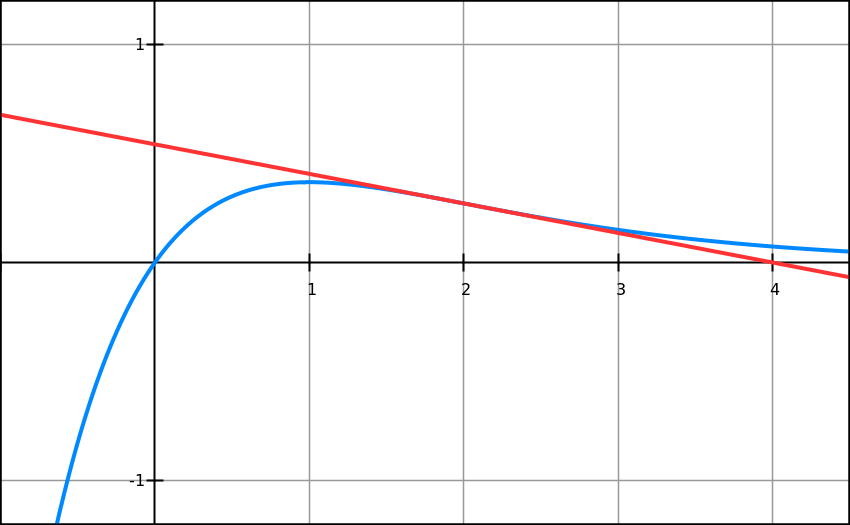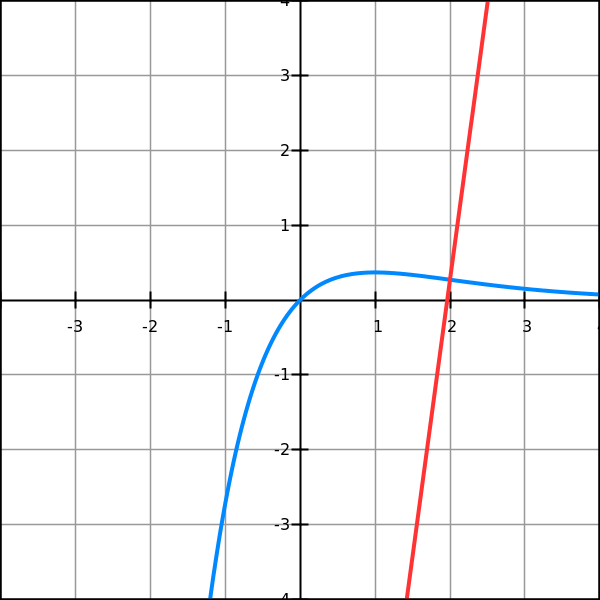## Thursday, January 12, 2017

### Unizor - Derivatives - Exercises 2

Notes to a video lecture on http://www.unizor.com

Derivatives - Exercises 2

Exercise 2.1
Given a function f(x)=x·e -x.
Find an equation of a tangential line that touches this function at pointx=2
y = −e−2·x + 4·e−2Exercise 2.2
Given a function f(x)=x·e-x.
Find all its maximum, minimum and inflection points.
f I(x) = e−x·(1−x)
f II(x) = e−x·(x−2)
Point x=1 is a point of local maximum.
Point x=2 is a point of inflection.
There is no point of local minimum.

Exercise 2.3
Given a functionf(x)=sin(x)+cos(x).
Find all intervals where it's monotonically increasing and intervals where it's monotonically decreasing.
Intervals of monotonic increasing:
[−3π/4+2πn, π/4+2πn]
Intervals of monotonic decreasing:
[π/4+2πn, 5π/4+2πn]

Exercise 2.4
Given a function f(x)=sin(x).
Find all inflection points of this function.
What are the first derivatives at these points?
x = π·n
First derivatives equal to 1 or −1

Exercise 2.5
Given a function f(x)=x·e-x.
Find an equation of a normal to its graph at point x=2
y = e2·x + 2·(e−2−e2)Exercise 2.6
Given a sufficiently smooth function f(x).
Find equations of a tangential line and a normal to its graph at pointx=x0